# JAC Class 10 Maths Solutions Chapter 13 Surface Areas and Volumes Ex 13.2

Jharkhand Board JAC Class 10 Maths Solutions Chapter 13 Surface Areas and Volumes Ex 13.2 Textbook Exercise Questions and Answers.

## JAC Board Class 10 Maths Solutions Chapter 13 Surface Areas and Volumes Exercise 13.2

Unless stated otherwise, take π = $$\frac{22}{7}$$

Question 1.
A solid is in the shape of a cone standing on a hemisphere with both their radii being equal to 1 cm and the height of the cone equal to its radius. Find the volume of the solid in terms οf π.
Solution:
The given solid is a combination of a cone and a hemisphere.
We have radius of the cone r = Radius of the hemisphere = 1 cm and height of the cone h = 1 cm.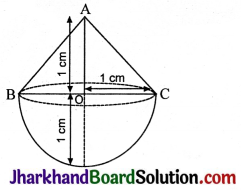∴ Volume of the solid = Volume of the cone + Volume of the hemisphere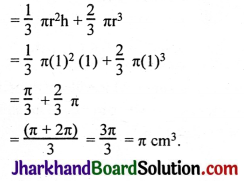Question 2.
Rachel, an engineering student, was asked to make a model shaped like a cylinder with two cones attached at its two ends by using a thin aluminium sheet. The diameter of the model is 3 cm and its length is 12 cm. If each cone has a height of 2 cm, find the volume of air contained in the model that Rachel made. (Assume the outer and inner dimensions of the model to be nearly the same.)
Solution:
d = 3 cm, AF = 8 cm = H, Ax = Fy = 2 cm = h, r = $$\frac{3}{2}$$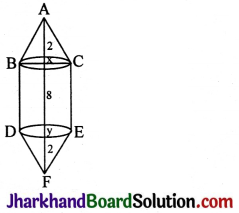Volume of the model = Volume of the cone ABC + Volume of the cylinder BCED + Volume of the cone DEF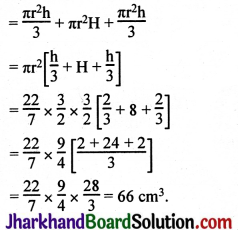Question 3.
A gulab jamun contains sugar syrup up to about 30% of its volume. Find approximately how much syrup would be found in 45 gulab jamuns, each shaped like a cylinder with two hemispherical ends with length 5 cm and diameter 2.8 cm.
Solution: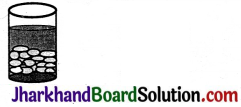Volume of 1 jamun = Volume of the cylinder + 2 × Volume of the hemisphere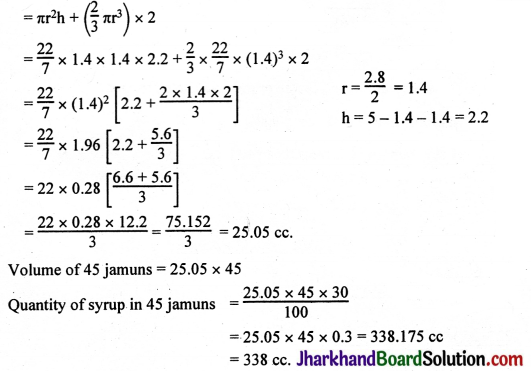Question 4.
A pen stand made of wood is in the shape of a cuboid with four conical depressions to hold pens. The dimensions of the cuboid are 15 cm by 10 cm by 3.5 cm. The radius of each of the depressions is 0.5 cm and the depth is 1.4 cm. Find the volume of wood in the entire stand.
Solution:Volume of wood in the stand = Volume of the cuboid – Volume of wood lost in making four conical depressions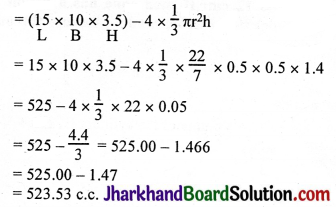Question 5.
A vessel is in the form of an inverted cone. Its height is 8 cm and the radius of its top, which is open, is 5 cm. It is filled with water up to the brim. When lead shots, each of which is a sphere of radius 0.5 cm are dropped into the vessel, one-fourth of the water flows out. Find the number of lead shots dropped in the vessel.
Solution:
Volume of water in the cone = $$\frac{\pi r^2 h}{3}$$
= $$\frac{1}{3} \times \frac{22}{7}$$ × 5 × 5 × 8 cc.
= $$\frac{22 \times 25 \times 8}{21}$$ c.c.
When some lead shots are dropped into the vessel, volume of the water that flows out= $$\frac{1}{4} \times \frac{22 \times 25 \times 8}{21}$$
= $$\frac{22 \times 25 \times 2}{21}$$ cc
This is equal to the volume of the lead shots dropped.
Volume of a lead shot = $$\frac{4}{3}$$πr3
Let the no. of lead shots dropped be x.
The volume of x lead shots = Volume of water overflown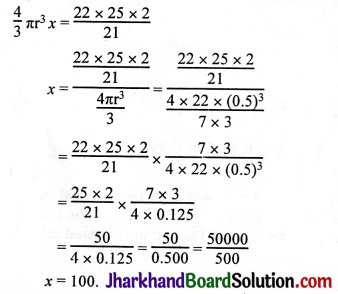Number of lead shots dropped = 100.Question 6.
A solid iron pole consists of a cylinder of height 220 cm and base diameter 24 cm, which is surrounded by another cylinder of height 60 cm and radius 8 cm. Find the mass of the pole, given that 1 cm3 of iron has approximately 8 g mass. (Use π = 3.14).
Solution:
R = 12, r = 4.
Common factor of 144 and 64 is 16 (HCF)
HCF of 220 and 60 is 20.
1 kg = 1000 gms.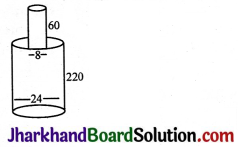Volume of the given solid = Volume of the bigger cylinder + Volume of the surmounted cylinder
= πR2H + πr2h
= 3.14 × 122 × 220 + 3.14 × 82 × 60
= 3.14 × 144 × 220 + 3.14 × 64 × 60
= 3.14 × 16 × 20(9 × 11 + 4 × 3)
= 3.14 × 320(99 + 12)
= 3.14 × 320 × 111 c.c.
Mass of the solid = Volume × density
= 3.14 × 320 × 111 × 8 gm
= $$\frac{3.14 \times 320 \times 111 \times 8}{1000}$$kg.
= $$\frac{3.14 \times 32 \times 888}{100}$$
= 3.14 × 32 × 8.88
= 892.26 kg.

Question 7.
A solid consisting of a right circular cone of height 120 cm and radius 60 cm standing on a hemisphere of radius 60 cm is placed upright in a right circular cylinder full of water such that it touches the bottom. Find the volume of water left in the cylinder, if the radius of the cylinder is 60 cm and its height is 180 cm.
Solution:
h = 180 (cylinder), H = 120 (Cone).
Volume of the solid given = Volume of the cone + Volume of the hemisphere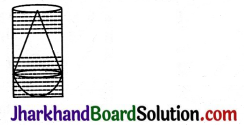Volume of water left in the cylinder = Volume of water in the cylinder – Volume of the solid immersed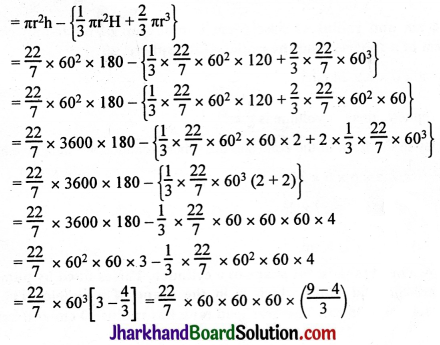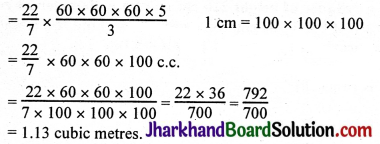Question 8.
A spherical glass vessel has a cylindrical neck 8 cm long, 2 cm in diameter; the diameter of the spherical part is 8.5 cm. By measuring the amount of water it holds, a child finds its volume to be 345 cm3. Check whether she is correct, taking the above as the inside measurements, and π = 3.14.
Solution:
r = $$\frac{8.5}{2}$$, R = $$\frac{2}{2}$$ = 1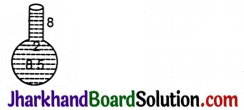Volume of water in the vessel = Volume of water in the spherical part + Volume of water in the cylindrical neck.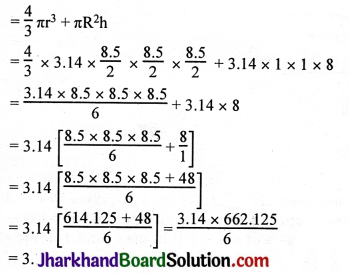= 3.14 × 110.354 = 346.51 cm3.